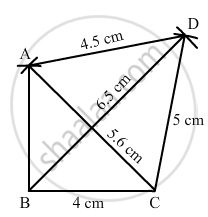Share

# Construct a Quadrilateral Abcd in Which Bc = 4 Cm, Ca = 5.6 Cm, Ad = 4.5 Cm, Cd = 5 Cm and Bd = 6.5 Cm. - Mathematics

Course
ConceptConstructing a Quadrilateral When Two Diagonals and Three Sides Are Given

#### Question

Construct a quadrilateral ABCD in which BC = 4 cm, CA = 5.6 cm, AD = 4.5 cm, CD = 5 cm and BD = 6.5 cm.

#### Solution

Steps of construction:

Step I: Draw BC = 4 cm .

Step II: With B as the centre and radius 6 . 5 cm, draw an arc .

Step III : With C as the centre and radius 5 cm, draw an arc to intersect the arc drawn in Step II at D .

Step IV: With C as the centre and radius 5 . 6 cm, draw an arc on the same side .

Step V : With D as the centre and radius 4 . 5 cm, draw an arc to intersect the arc drawn in Step IV at A .

Step VI: Join BA, AC, DA, BD and CD to obtained the required quadrilateral .Is there an error in this question or solution?

#### APPEARS IN

RD Sharma Solution for Mathematics for Class 8 by R D Sharma (2019-2020 Session) (2017 to Current)
Chapter 18: Practical Geometry (Constructions)
Ex. 18.2 | Q: 6 | Page no. 6
Solution Construct a Quadrilateral Abcd in Which Bc = 4 Cm, Ca = 5.6 Cm, Ad = 4.5 Cm, Cd = 5 Cm and Bd = 6.5 Cm. Concept: Constructing a Quadrilateral - When Two Diagonals and Three Sides Are Given.
S